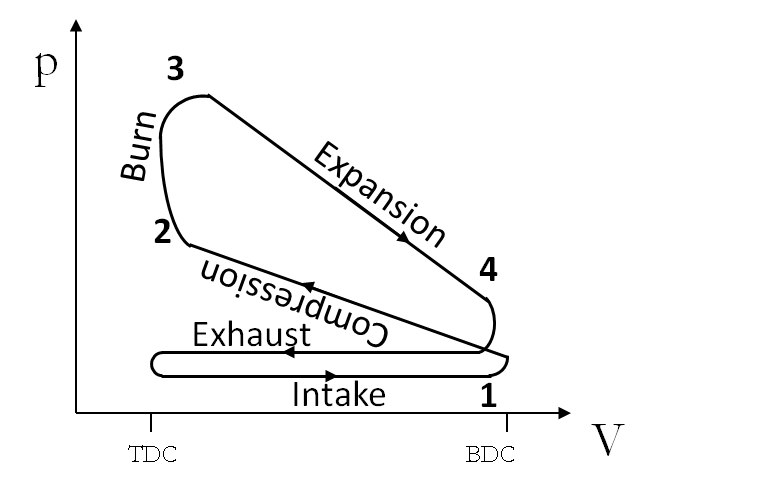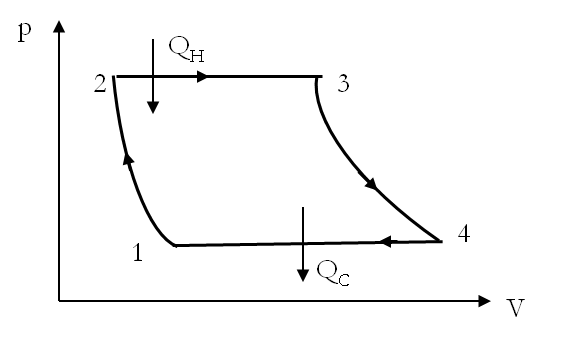## Section25.5Real Engines

### Subsection25.5.1The Efficiency of a Real Heat Engine

Real heat engines also transform heat into work. Real engines lack the ideal condition envisioned in the Carnot cycle which causes the efficiency of real engines to be less than that of a Carnot engine working between the same two temperature baths.

\begin{equation} \eta_{\text{real}} \lt \eta_{\text{Carnot}} = 1- \frac{T_C}{T_H}\tag{25.5.1} \end{equation}

We obtain the efficiency of real engines from the work done $W$ output of the engine for unit amount of heat absorbed, $Q_{H}\text{.}$

\begin{equation} \eta_{\text{real}} \ne 1- \frac{T_C}{T_H}, \text{but instead}, \eta_{\text{real}} = \frac{W}{Q_{H}}.\tag{25.5.2} \end{equation}

The efficiency of the Carnot engine serves as an upper bound on the efficiency of all engines, and is often used as guide for rough calculations.

A power plant uses a steam engine to produce $300\text{ MW}$ of electric power. The steam in the power plant is heated by burning natural gas that releases $1000\text{ MJ}$ of heat per second. The steam enters the turbine at a temperature of $660\text{K}$ and is discharged to the condenser at $310\text{K}\text{.}$ Cold water runs through the condenser to cool the hot water there thus removing the heat at lower temperature.

(a) What is the actual efficiency of the power plant, and how does it compare with the maximum efficiency possible?

(b) If the temperature of the running water through the condenser rises by $10^{\circ}\text{C}\text{,}$ what is the rate at which water must be pumped through the condenser?

Hint

(a) Actual efficiency is based on energy and the ideal efficiency can be obtained from absolute temperatures. (b) Use $mc\Delta T$ to account for heat carried away by water.

(a) 30%, 53%, (b) $1.67\times 10^4\ \text{kg/s}\text{.}$

Solution 1 (a)

(a) The actual efficiency is given by the ratio of work produced to the heat taken in. The energy figures in the question are given as powers, therefore, we look at the energies in one second. Hence,

\begin{equation*} \eta_{\text{real}} = \frac{W}{Q_{H}} = \frac{300\ \text{MJ/s}\times 1 \text{s}}{1000 \ \text{MJ/s}\times 1 \text{s}} = 0.3 = 30%. \end{equation*}

The maximum efficiency is given by the ideal Carnot engine formula.

\begin{equation*} \eta_{\text{max}} = 1-\frac{T_C}{T_H} = 1- \frac{310\ \text{K}}{660\ \text{K}} = 0.53 = 53%. \end{equation*}
Solution 2 (b)

(b) The running water must remove $Q_C$ amount of heat per second. Based on the given data, the value of $Q_C$ is:

\begin{equation*} Q_C = Q_H - W = 1000\ \text{MJ} - 300\ \text{MJ} = 700\ \text{MJ}. \end{equation*}

Let $x$ be the rate in $kg/s$ of the water pumped through the condenser to remove $Q_C$ amount of heat per second. Then we must have

\begin{equation*} x c\Delta T = \frac{Q_C}{1\ \text{sec}}\ \ \text{(per second)} \end{equation*}

Therefore,

\begin{equation*} x = \frac{700\times 10^6\ \text{J/s}}{4186\ (\text{J/kg.C})\times 10\text{C}} = 1.67\times 10^4\ \text{kg/s}. \end{equation*}

A coal power plant consumes $100,000\text{ kg}$ of coal per hour and produces $500\text{ MW}$ of power. What is the efficiency of the power plant?

Data: heat of combustion of coal is 30 MJ/kg.

Hint

$0.6$ or $60\%\text{.}$

Solution

From the given data we find that energy used per sec is

\begin{equation*} \frac{ 100,000\text{ kg} \times 30\text{ MJ/kg} }{3600\text{ s}} = 833.33\test{ MJ/s}. \end{equation*}

The energy produced per sec is $500\text{ MW} = 500\text{ MJ/s}\text{.}$ Therefore, efficiency of the power plant is

\begin{equation*} \frac{500\text{ MJ/s}}{833.33\test{ MJ/s}} = 0.60. \end{equation*}

### Subsection25.5.2Internal Combustion Engine

Internal combustion engine of automobiles based on Otto cycle is perhaps the most widely used engine in the world. The idealized Otto cycle is shown in Figure 25.5.3. The Otto cycle consists of the following steps on a system consisting of a mixture of air and fuel.

1. Intake stroke: Intake valve is opened and piston retracts sucking in air and gasoline vapor at constant pressure of 1 atmosphere.
2. Adiabatic compression: The intake valve is closed and the piston is pushed rapidly so that the process is adiabatic compression. Both $p$ and $T$ increase in this step.
3. Combustion and power stroke: In gasoline engines, a spark plug ignites the mixture of gasoline vapor and air under pressure. The power stroke is rapid enough to be adiabatic. If a process is rapid, there is no time for the system to exchange heat with outside and hence adiabatic.
4. Exhaust: Exhaust valve is opened and high pressure combustion products are exhausted.Figure 25.5.3. Otto cycle of an internal combustion engine. Here TDC and BDC stand for top dead center and bottom dead center. At TDC, the piston is at the top and at BDC it is at the bottom. Work done by the engine in one cycle is the area enclosed by the cycle.

The efficiency for the Otto cycle, derived below, is similar to that of Carnot cycle, except the fact that here we do not have a constant temperature bath.

\begin{equation*} \eta_{\text{Otto;Ideal}} = 1- \frac{T_4}{T_3} = 1- \frac{T_1}{T_2} \end{equation*}

where $T_1$ is the temperature of the chamber at the beginning of the adiabatic compression stroke and $T_2$ the temperature at the end of the compression.

(Calculus) Derivaiton of the Efficiency of the Otto Engine

Efficiency of any heat engine is the work produced over heat input. Since we have a cyclic process for the material occupied in the space of the cylinder, work done is equal to the difference of heat input in the combustion and the heat exhausted after the power stroke. Both the heat produced and heat exhausted are constant volume processes and not constant temperature processes. Therefore, we use $Q_{in}$ and $Q_{out}$ for them in place of $Q_H$ and $Q_C$ used above for the Carnot engine.

Heat is taken in by the engine in the ignition phase raising the temperature from $T_2$ to $T_3\text{,}$ and heat is rejected by the engine when the exhaust valve opens which takes the temperature from $T_4$ to $T_1\text{.}$ Assuming specific heat at constant volume to be independent of temperature we can obtain the following expressions for the heat in and heat out.

\begin{align*} \amp Q_{in} = \int_{T_2}^{T_3}nC_v dT \approx nC_v\left( T_3 - T_2 \right)\\ \amp Q_{out} = \int_{T_1}^{T_4}nC_v dT \approx nC_v\left( T_4 - T_1 \right) \end{align*}

Therefore, the efficiency of the Otto engine is

\begin{equation*} \eta_{\text{Otto}} = 1- \frac{Q_{out}}{Q_{in}} = 1 - \left( \frac{T_4 - T_1}{T_3 - T_2} \right) \end{equation*}

This formula can be further simplified if we assume the ideal gas behavior for the gasoline/air mixture. Efficiency is then called the ideal efficiency, $\eta_{Ideal}\text{.}$ For ideal gas, two states on an adiabatic path are related by the following useful formula.

\begin{equation*} TV^{\gamma-1} = \text{Constant}. \end{equation*}

Thus during the adiabatic compression and power stroke steps we have the following relations.

\begin{align*} \amp \text{Adiabatic compression:}\ \ T_1 V_1^{\gamma-1} = T_2 V_2^{\gamma-1}\\ \amp \text{Power stroke:}\ \ T_3 V_3^{\gamma-1} = T_4 V_4^{\gamma-1} \end{align*}

In addition we have the following relations because the other two steps are constant volume processes.

\begin{align*} \amp V_2 \approx V_3,\\ \amp V_1\approx V_4. \end{align*}

Using these conditions we arrive at the following relation among temperatures at various points of the Otto cycle for an ideal gas.

\begin{equation*} \frac{T_1}{T_4} = \frac{T_2}{T_3} \end{equation*}

This helps simplify the efficiency formula for the Otto cycle to the following.

\begin{equation*} \eta_{\text{Otto;Ideal}} = 1- \frac{T_4}{T_3} = 1- \frac{T_1}{T_2} \end{equation*}

where $T_1$ is the temperature of the chamber at the beginning of the adiabatic compression stroke and $T_2$ the temperature at the end of the compression. The ideal efficiency formula looks a lot like the Carnot engine formula, except here there are no constant temperature heat baths.

### Subsection25.5.3Steam Engine

You have probably heard of the story about James Watt having invented the steam engine after watching a kettle boil. This story told and retold in elementary schools is very likely fiction since steam engine was invented before James Watt. Newcomen engine invented in 1712 was perhaps the first true steam engine. In a Newcomen engine steam is allowed in a cylinder which raises a piston thus doing a work on the external agent. The cylinder is the sprayed with cold water condensing steam inside and creating a partial vacuum which brings the piston back to the original position thus completing the cycle which can be repeated indefinitely. The moving piston could be used to move anything not just water from mines.

Around 1760's while working as an instrument maker at the University of Glasgow, James Watt was asked to repair the University's model Newcomen steam engine. He found the cooling of the steam for retracting the piston resulted in parts that had to be reheated again and thus constituted an unnecessary loss of energy. He introduced a second cylinder for cooling the steam and made some other modifications that led to $75\%$ increase in efficiency. He patented this and other improvements to the Newcomen engine in 1769. By 1783 the Watt engines had replaced all the Newcomen engines. The more efficient and therefore more cost-effective Watt engine made the consolidation of small cottage industries into more efficient larger factories, a key ingredient of the Industrial Revolution, possible.

The steam engine is an external combustion engine where heat from burning coal, wood or other fossil fuel, or energy from nuclear disintegrations is used to heat water in a boiler. To maximize the transfer of heat the boiler has to be designed properly. The superheated steam is then sent through an intake valve into a chamber for pushing on a piston in case of a reciprocating type engine or through a turbine which has rotating blades for a turbine engine. After that the intake valve is closed and exit valve is opened. The wet steam is pushed into the condenser which cools the wet steam into water. The water is then pumped into the boiler thus completing the cycle. Just as before, the net work done divided by the heat intake gives the efficiency of the engine. The cycle for water in the steam engine, also called the Rankine cycle, is displayed in Figure 25.5.4.Figure 25.5.4. The Rankine cycle of a steam engine. Steps are 1-2: pumping into boiler, 2-3: boiling, 3-4: power stroke, and 4-1: condensing.

The thermodynamics steps in the Rankine cycle are:

1. [$1\rightarrow 2:$] Adiabatic compression of water from condenser to the pressure of the boiler. Work $W_{12}$ is done on the system.
2. [$2\rightarrow 3:$] Heating of water at constant pressure. Water is heated to a temperature $T_H$ beyond the boiling point creating superheated steam. Heat $Q_{in}$ is taken in by the system and work $W_{23}$ is done.
3. [$3\rightarrow 4:$] Adiabatic expansion of steam in the power stroke. Work $W_{34}$ is done on the system.
4. [$3\rightarrow 4:$] Cooling the wet steam into saturated water at constant pressure at temperature $T_C$ in the condenser.

The Efficiency of a Steam Engine

By energy conservation, work output is equal to $Q_{in}- Q_{out}\text{.}$ Therefore, the efficiency of a steam engine is given as follows.

\begin{equation*} \eta = \frac{Q_{in}- Q_{out}}{Q_{in}} \end{equation*}

The processes where heat exchange with the system takes place are constant pressure processes. We have learned that heat exchange $Q$ at constant pressure is equal to the enthalpy change $\Delta H$ of the system.

\begin{equation*} \left. Q\right|_{\text{const p}} = \Delta H. \end{equation*}

Therefore heat $Q_{in}$ and $Q_{out}$ in the Rankine cycle are

\begin{align*} \amp Q_{in} = |H_3-H_2| = |U_3+p_3V_3 -U_2-p_2V_2| \ \ \ (p_2=p_3)\\ \amp Q_{out} = |H_1-H_4| = |U_1+p_1V_1 -U_4-p_4V_4| \ \ \ (p_1=p_4) \end{align*}

Therefore, the efficiency of a Rankine engine can be written in terms of enthalpy of the states.

\begin{equation*} \eta_{\text{Rankine}} = 1 - \frac{|H_1-H_4|}{|H_3-H_2|} \end{equation*}

The enthalpy of states 1 and 2 are nearly equal since in the pumping not much energy is added to the system.

\begin{equation*} H_1\approx H_2. \end{equation*}

Therefore to figure out the efficiency of a steam engine we need to know enthalpy for three states only.

\begin{equation*} \eta_{\text{Rankine}} = 1 - \frac{|H_4-H_1|}{|H_3-H_1|} \end{equation*}